Name:    Chapter 9 Test

Multiple Choice
Identify the choice that best completes the statement or answers the question.

1.

There are 16 sixth graders, 11 seventh graders, and 9 eighth graders in a gym class. The gym teacher randomly selects one student to collect balls. In how many ways can choosing not a seventh grader occur?
 a. 11 ways c. 25 ways b. 20 ways d. 36 ways

2.

The possible rolls on a number cube are 1, 2, 3, 4, 5, and 6. Which word best describes the likelihood of rolling not an 8?
 a. equally likely c. impossible b. certain d. likely

Use the spinner to determine the theoretical probability of the event.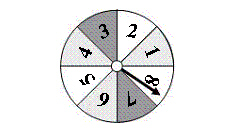3.

Spinning a 3
 a.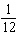c.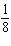b.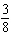d.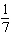4.

Spinning a number less than 5
 a.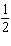c.b.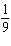d.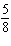5.

Spinning a number less than 11
 a. 1 c.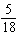b.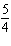d.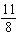6.

Spinning an even number
 a.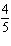c.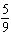b.d.7.

Spinning a multiple of 4
 a.c.b.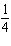d.8.

You shuffle a deck of 26 cards representing the letters of the alphabet. You randomly select a card from the deck. What is the theoretical probability that the card represents one of the letters in the word “mathematics”?
 a.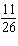c.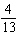b.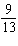d.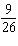9.

The partial tree diagram shows some of the outcomes of flipping a coin four times. How many possible outcomes are there in all?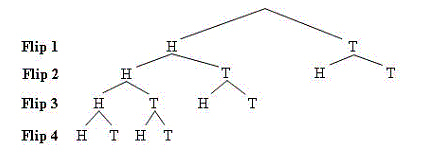a. 16 c. 12 b. 8 d. 4

10.

You write the numbers 1 through 9 on separate pieces of paper and put them in a hat. Then you draw the numbers one at a time without replacing them. What is the probability that you draw the numbers 1 through 9 in order?
 a.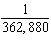c.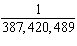b.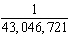d.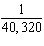11.

You have 8 pairs of socks in the dryer. Only one pair is black. You randomly choose two of the socks. What is the probability that you got both of the black socks?
 a.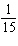c.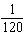b.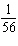d.Numeric Response

1.

There are 98 lettered tiles in a board game. You randomly draw the tiles shown. How many of the 98 lettered tiles would you expect to be vowels?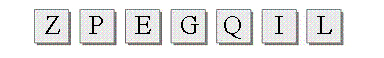2.

A baseball team has won 50% of its games so far. There are 20 regular season games left. Predict how many of these games the team will win.

You randomly choose one of the tiles shown below. Find the favorable outcomes of the event.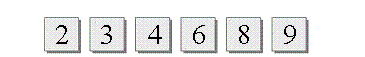1.

Choosing a number less than 4

You spin both spinners. Find the probability of the events.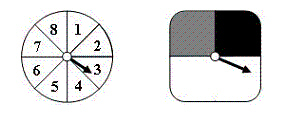2.

Spinning a 5 and not spinning white